## Wednesday, June 13, 2007

### 070612_Voronoi3D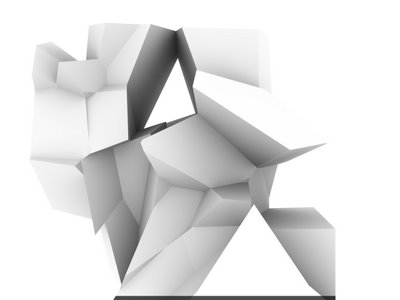Interesting - I always thought I subconsciously "avoided" to work around 3D Voronoi partly for preconception as "over trendy", partly becausenever solving any other problem for me other than beautifull intricate cellular packing - I finally had to write my own code to generate a 3D Voronoi as an under layer piece of code for an other design tool application.
The structure of the code written in rhinoscript DOESN"T REQUIRE THE NEED OF QHull data set; the code somehow "happened" to become a 3D Voronoi while originally looking at a different purpose - looking at shapes describe through some kind of wireframe in tension based on locator in 3D space - the striking similarity of the first result finally forced me to spend few more extra hours to force the code to output a more mathemically purist version of the 3D Voronoi... finally or simply once more "never say never"...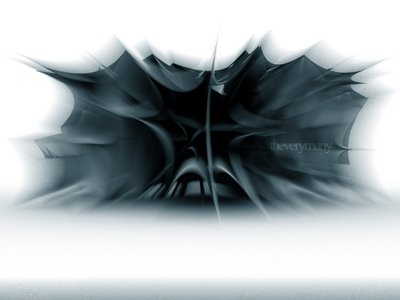"Mathematically purist" - well within our more and more complex understanding of our contemporary society, the word "purist" can only be a very narrow and dangerous call - and I do apologize for that... my interpretation at the time was a qualificatif for simply following straight forward the steps of the Voronoi 3D geometrical description... sorry - no funky mathematical algorythm...
Though staying within rhino - a CAAD software - and write your own tool allows I believe to be in control of your code and therefore be able to manipulated away from its primarly algorythm toward a design tool - like with the 2D version for exemple...

First "delirium tremens"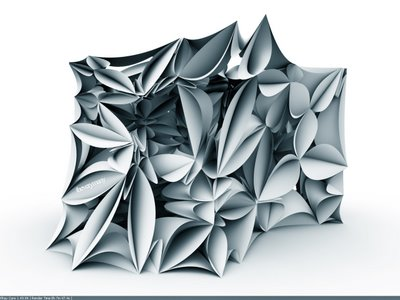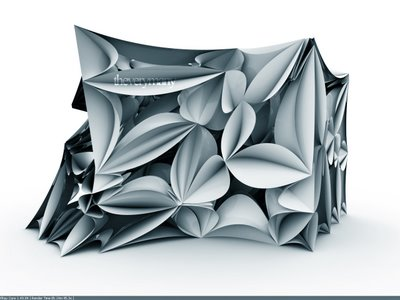Labels: ,

## Tuesday, January 23, 2007

### 070122_Polytop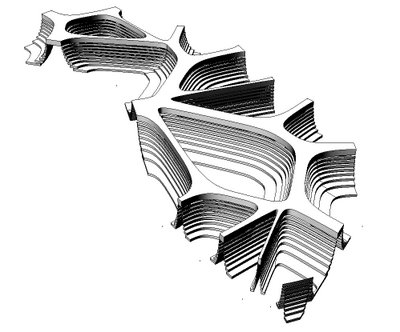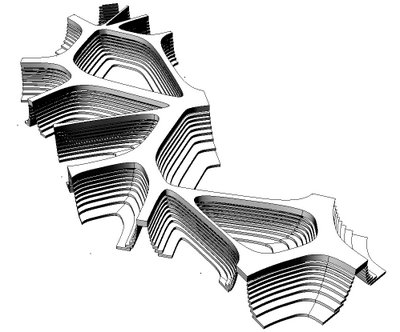"POLYTOP" / Marc Fornes & theverymany

"Polytop" is a RANGE of a mass customized coffee tables: each table is different but similar without changing the cost of production: the generative automaton processs for each single entity is starting from the same base frame (according to material and standart sheet size); the code is first plotting a number of pts (according to user specifications) to create a pt cloud onto which is running a customized 2.5D Voronoi; here speculating further onto the use of Vornoi diagrams within the field of design, theverymany is looking at optimization within the production process (more of problem caring than problem solving): only 3 axis require for the CNC cut (though the use of a taper tool allows smoother transitions), reducion of the amout of cuts (each cut is used on both side of the line) and reduction of waste of material : within the production process, every cut out is used to produced an n+2 layer within the vertical section: with one sheet of material you can therefore produce at least 3 to 5 layers.

TABLE (Wikipedia.org)

Etymology: the term "table" is derived from a merger of French table and Old English tabele, ultimately from the Latin word tabula, "a board, plank, flat piece". In Late Latin, tabula took over the meaning previously reserved to mensa (preserved in Spanish mesa "table"). In Old English, the word replaced bord for this meaning.

POLYTOPE (Mathworld.com)

The word polytope is used to mean a number of related, but slightly different mathematical objects.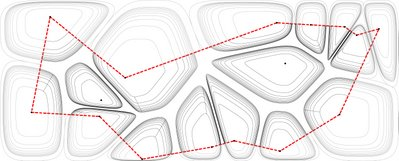VORONOI (Mathworld.com)

A polygon whose interior consists of all points in the plane which are closer to a particular lattice point than to any other. The generalization to dimensions is called a Dirichlet region, Thiessen polytope, or Voronoi cell.

Labels: ,

## Monday, January 22, 2007

### 070122_rh4_Voronoi&Arcs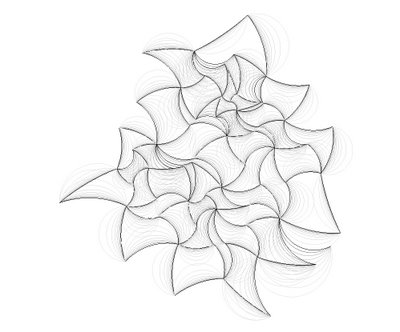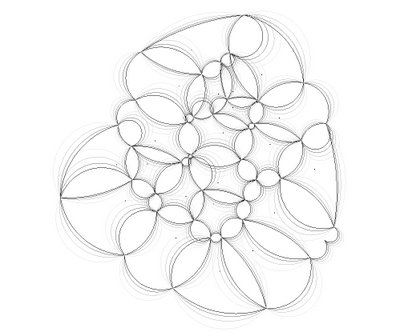"VORONARC"

Those two drawings are part of a speculative research onto Voronoi and Arcs; the serie is looking beyond the trend of Voronoi diagrams and their most common graphical output as cellular aggragates (now used within every architectural school!); here encoded arcs are used to illustrate possible structural moments within each cell boundaries segments...

ARC (wikipedia.org)

In Euclidean geometry, an arc is a closed segment of a differentiable curve in the two-dimensional plane; for example, a circular arc is a segment of a circle. If the arc segment occupies a great circle (or great ellipse), it is considered a great-arc segment.

The length of a circular arc of a circle with radius r and subtending an angle θ (measured in radians) with the circle centre, equals θr. For an angle α measured in degrees, the size in radians is given by (α/180°) × π, and so the arc length equals then (α/180°)πr.

Tips'n tricks : (ie: David Rutten's Rhinoscript101 on Arc)

Function AddArcDir(ByVal ptStart, ByVal ptEnd, ByVal vecDir)
Dim vecBase : vecBase = Rhino.PointSubtract(ptEnd, ptStart)
If Rhino.VectorLength(vecBase) = 0.0 Then Exit Function
If Rhino.IsVectorParallelTo(vecBase, vecDir) Then Exit Function
vecBase = Rhino.VectorUnitize(vecBase)
vecDir = Rhino.VectorUnitize(vecDir)
Dim vecBisector : vecBisector = Rhino.VectorAdd(vecDir, vecBase)
vecBisector = Rhino.VectorUnitize(vecBisector)
Dim dotProd : dotProd = Rhino.VectorDotProduct(vecBisector, vecDir)
Dim midLength : midLength = (0.5 * Rhino.Distance(ptStart, ptEnd)) / dotProd
vecBisector = Rhino.VectorScale(vecBisector, midLength)
End Function

Labels: , ,

## Monday, August 07, 2006

### Rh4_060807_VORNOI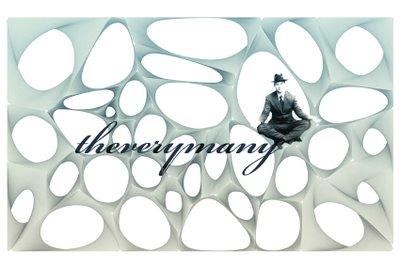In mathematics, a Voronoi diagram, named after Georgy Voronoi, also called a Voronoi tessellation, a Voronoi decomposition, or a Dirichlet tessellation (after Lejeune Dirichlet), is special kind of decomposition of a metric space determined by distances to a specified discrete set of objects in the space, e.g., by a discrete set of points.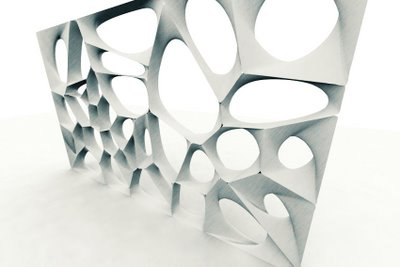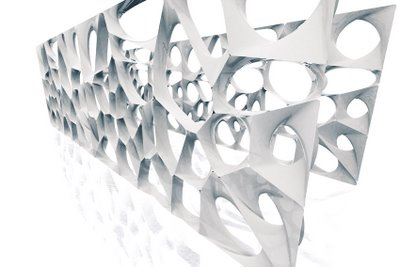DEFINITION
For any (topologically) discrete set S of points in Euclidean space and for almost any point x, there is one point of S closest to x. The word "almost" is occasioned by the fact that a point x may be equally close to two or more points of S.

If S contains only two points, a and b, then the set of all points equidistant from a and b is a hyperplane — an affine subspace of codimension 1. That hyperplane is the boundary between the set of all points closer to a than to b, and the set of all points closer to b than to a. It is the perpendicular bisector of the line segment from a and b.

In general, the set of all points closer to a point c of S than to any other point of S is the interior of a (in some cases unbounded) convex polytope called the Dirichlet domain or Voronoi cell for c. The set of such polytopes tessellates the whole space, and is the Voronoi tessellation corresponding to the set S. If the dimension of the space is only 2, then it is easy to draw pictures of Voronoi tessellations, and in that case they are sometimes called Voronoi diagrams.

The dual for a Voronoi tessellation is the Delaunay triangulation for the same set of points S.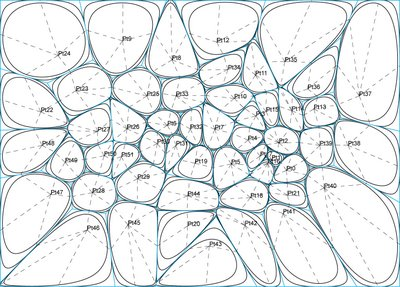GENERALIZATIONS
Voronoi cells can be defined for metrics other than Euclidean. However in these cases the Voronoi tessellation is not guaranteed to exist (or to be a "true" tessellation), since the equidistant locus for two points may fail to be subspace of codimension 1, even in the 2-dimensional case.

Voronoi cells can also be defined by measuring distances to areas rather than to points. These types of Voronoi cells are used in image segmentation, optical character recognition and other computational applications. In materials science, polycrystalline microstructures in metallic alloys are commonly represented using Voronoi tessellations.
ie WIKIPEDIA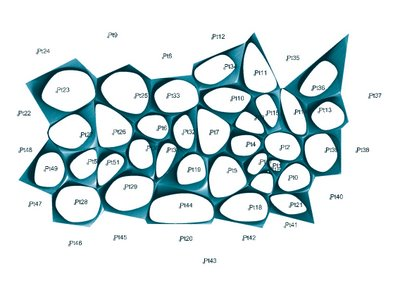VORNOI CODE (from challenge last week at the office + Rassul...)
The Voronoi core has been developped by David Rutten (reconstructivism.net)

Function VoronoiPolygon(index, datSet, BBox)
' this function creates a voronoi cell for agiven point in a set of points
' should probably be optimized so that it only tests points near the samplepoint
VoronoiPolygon = Null

Dim midPt, arrPt, vecDir(1)
Dim ptS(2), ptE(2)
Dim ChordLength, Border
Dim brdLines(), i, N
ReDim brdLines(UBound(datSet)-1)
ChordLength = Rhino.Distance(BBox(0), BBox(2))

arrPt = datSet(index)
N = 0

For i = 0 To UBound(datSet)
If i <> index Then
midPt = Array((datSet(i)(0) + datSet(index)(0))/2, _
(datSet(i)(1) + datSet(index)(1))/2, _
0)
vecDir(0) = -(datSet(i)(1)-datSet(index)(1))
vecDir(1) = datSet(i)(0)-datSet(index)(0)
vecDir(0) = vecDir(0)/Rhino.Distance(datSet(i), datSet(index))*ChordLength
vecDir(1) = vecDir(1)/Rhino.Distance(datSet(i), datSet(index))*ChordLength
ptS(0) = midPt(0)+vecDir(0)
ptS(1) = midPt(1)+vecDir(1)
ptS(2) = 0
ptE(0) = midPt(0)-vecDir(0)
ptE(1) = midPt(1)-vecDir(1)
ptE(2) = 0
N = N+1
End If

Next

Border = Rhino.AddPolyline(Array(Array(BBox(0)(0)-10, BBox(0)(1)-10, 0), _
Array(BBox(1)(0)+10, BBox(1)(1)-10, 0), _
Array(BBox(2)(0)+10, BBox(2)(1)+10, 0), _
Array(BBox(3)(0)-10, BBox(3)(1)+10, 0), _
Array(BBox(0)(0)-10, BBox(0)(1)-10, 0)))
Rhino.UnselectAllObjects
Rhino.SelectObjects brdLines
Rhino.SelectObject Border
Rhino.Command "-_CurveBoolean _DeleteInput=No _CombineRegions=No " & _
Rhino.Pt2Str(Array(datSet(index)(0),datSet(index)(1),datSet(index)(2))) & _
" _Enter", vbFalse
' delete lines
Rhino.UnselectAllObjects
Rhino.SelectObjects brdLines
Rhino.Command "-_Delete"

VoronoiPolygon = vbTrue
End Function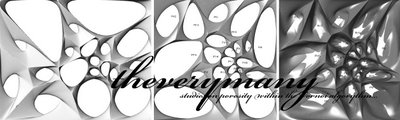Labels: